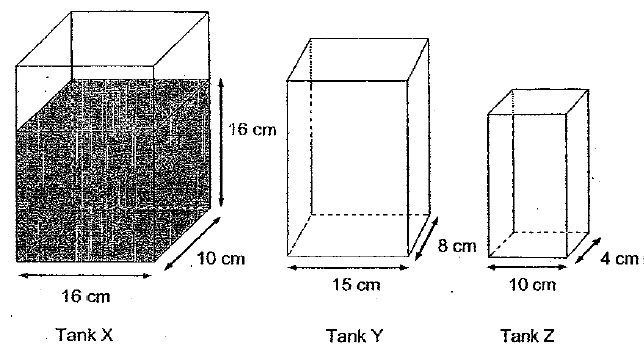# QuestionTank X is filled with water to a height of 16cm. The water in Tank X is poured into 2 rectangular tanks, Tank Y & Z. such that the heights of water in the 3 tanks are equal. Find the volume of water out of Tank X.

My answer for this Q is 1280 cm3 but the correct answer should be 1600 cm3. which part that i miss out? thank you .

16 x 10 x 16 = 2560
height x (16 x 10 + 15 x 8 + 10 x 4) = height x 320 ——– 2560
height ——- 2560/320 = 8
16 x 10 x 8 = 1280

Ans : 1280 cubic cm.